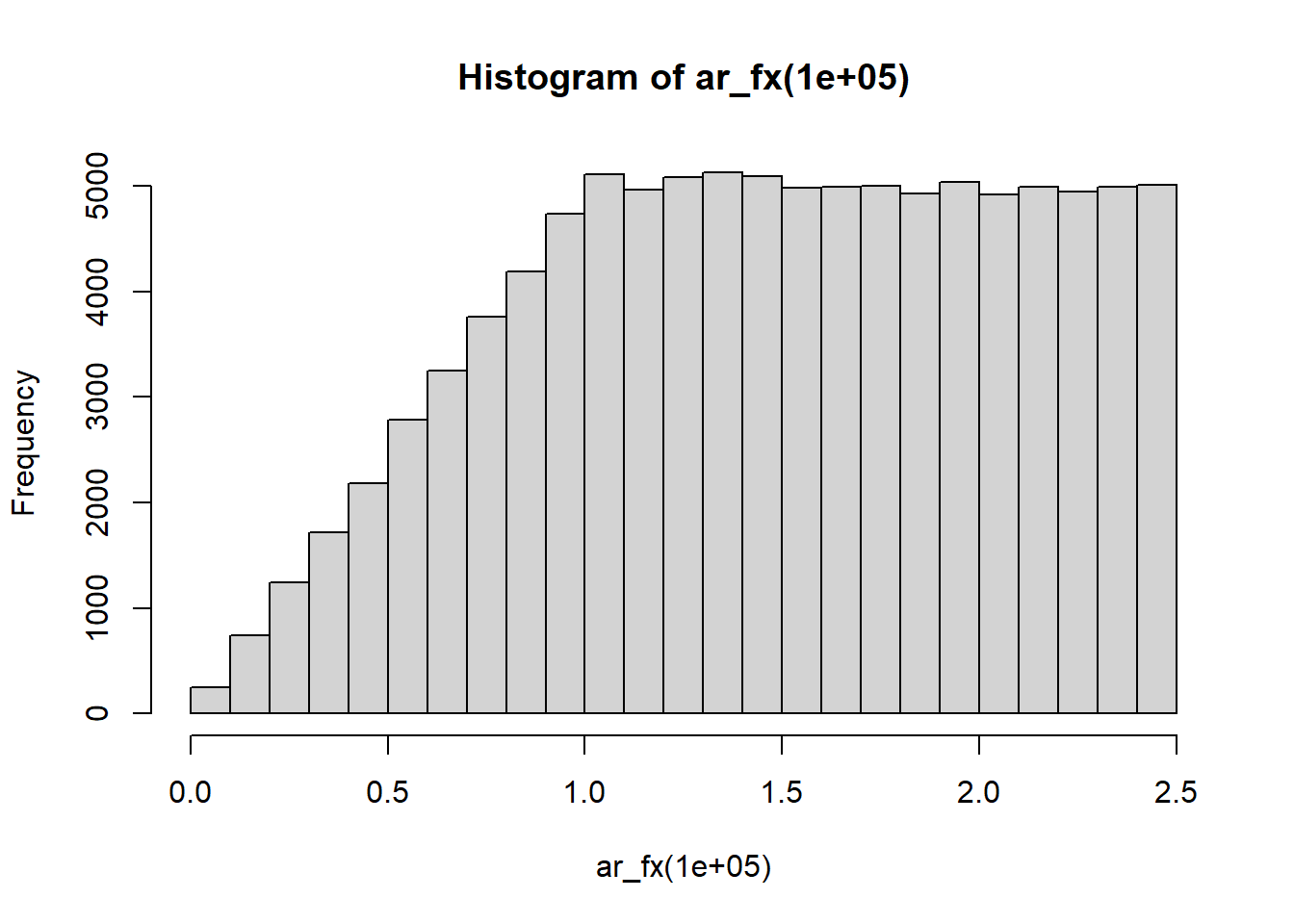#accept reject method
#n is the number of random values you want from the distribution f(x)
#accept reject method
ar_fx <- function(n){
generate <- NA  #empty vector for generated values
i = 1 #counter
while(i < n+1){ #i < n+1 = n
x <- runif(1) #for use in inverse transform of h(x)
u <- runif(1) # the uniform distribution
if(x < 4/25){  #f(x)/ch(x) for f(x) = .5x only uses h(x) from the range 0 to 4/25
generate[i] <- sqrt(x)*(2.5) #because u will always be less than 1 all x < 4/25 are accepted.
i = i + 1
}
else{
x <- sqrt(x)*5/2
if(u < x^-1){
generate[i] <- x
i = i + 1
}
#note that if u > x^-1 then the x rejected

}
}
return(generate)

}
hist(ar_fx(100000))